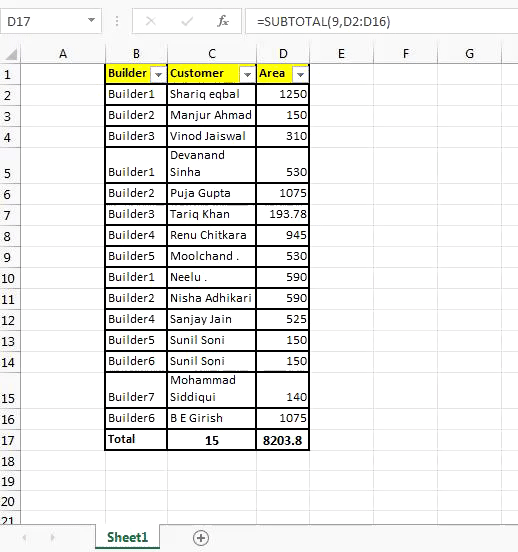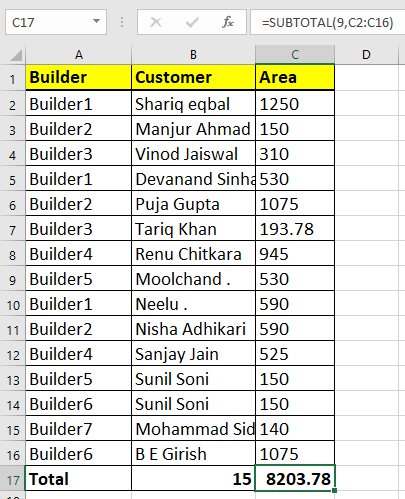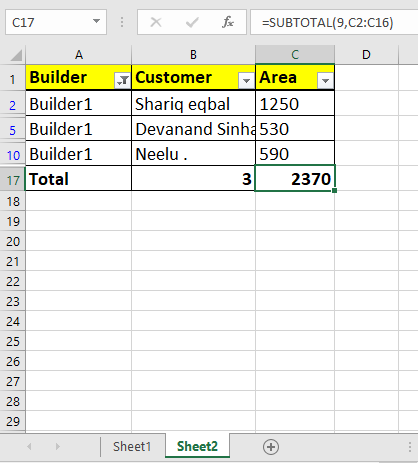# How to use the SUBTOTAL Function in Excel

The biggest problem with SUM, AVERAGE, COUNT and other NUMBER functions is, that they include rows that are hidden by filter. They don’t work correctly when we want to sum, count, or do any other number task only on visible cell. Here steps in the SUBTOTAL function of Excel.Syntax of SUBTOTAL

=SUBTOTAL(function_number, ref1, [ref2]...)

Function_number: Subtotal supports various number function. To easily access them, it uses function number. Below is the table of function number with corresponding functions.

#### EXAMPLE TABLE

 Function Number To Include Manually Hidden Rows Function Number To IgnoreManually Hidden Rows Function 1 101 AVERAGE 2 102 COUNT 3 103 COUNTA 4 104 MAX 5 105 MIN 6 106 PRODUCT 7 107 STDEV 8 108 STDEVP 9 109 SUM 10 110 VAR 11 111 VARP

Ref1 : The named range or reference of numbers.
[ref2] : optional named range or reference range to numbers.

Lets see an example:

#### SUBTOTAL Example

I have this table of real state. Now I want to add a totals row but it should show me sum of visible cell only when I apply a filter.

Write this formula in the end of table or the top. Your choice…

=SUBTOTAL(9,C2:C16)Now whenever you will apply a filter, only visible cells total will be shown.So yeah guys, SUBTOTAL is very useful when you create interactive dashboards. Let me know which function you use most of subtotal in comments section below. And don’t forget ask you doubts, they are most important.

Popular Articles:

50 Excel Shortcuts to Increase Your Productivity

How to use the VLOOKUP Function in Excel

How to use the COUNTIF function in Excel 2016

How to Use SUMIF Function in Excel

Terms and Conditions of use

The applications/code on this site are distributed as is and without warranties or liability. In no event shall the owner of the copyrights, or the authors of the applications/code be liable for any loss of profit, any problems or any damage resulting from the use or evaluation of the applications/code.